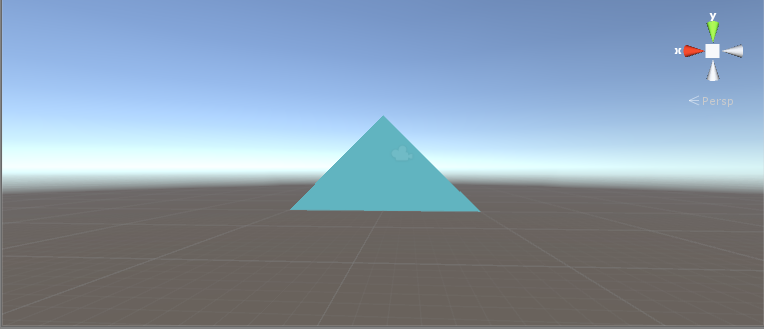#### Procedural Mesh in Unity, Part-1 Lets Draw A Triangle

31 Aug 2017 | Sujay#### What is a Procedural Mesh:

It is a method of algorithmically generating mesh geometry. Each vertex on the mesh is generated based on a set of rules in the algorithm. The mesh generated is usually based on mathematical equation.

#### The basics:

If you are exploring about procedural meshes, you probably know how computer graphics work. Basically your computer draws a set of vertices and starts to connect them to form triangles. After this the triangles undergo a set of shading process (which you can learn more here) which involves quite a few mathematical calculations. These set of triangles together appear as a 3 dimensional object on your screen. If you look closely, the basic shapes in Unity such as plane, cube, cylinder etc. all are made out of triangles. These models can be created using 3D modelling software like Blender, Autodesk Maya etc. But today, lets learn the art of creating them PROGRAMATICALLY.

#### Lets draw a basic triangle in Unity:

• Open Unity, and add a empty gameobject to the scene.
• To the new gameobject, add a meshfilter and a mesh renderer.
• In the mesh renderer, go ahead and add a suitable material.
• You can use default diffuse shader. Now add a new script to the gameobject.
• Lets name it as drawMesh.cs. Lets start adding the code.

Save the script, and play the game in Unity editor. Ta-da, you have a triangle in your scene!!

Let me explain what exactly has happened here.

The first block of the code involves declaring the variables for mesh and meshfilter. Next, in Start() function we create a new mesh, and we get the meshfilter from the gameobject and assign the newly created mesh to the meshfilter.

The drawTriangle function draws the triangle. To draw a triangle in a 3D space, first we need 3 points called vertices. We create an array for storing 3 vertices(VerteicesArray). We also need to tell Unity in which order it should use the given vertices to draw the triangle. To do this we need a array of integers(trianglesArray).

We first choose 3 vertices in 3d space. I have chosen all 3 vertices in XY plane. We store them in the array. Next, we need to give the order of vertices for triangle rendering. It is important to give the order of the vertices in clockwise. The contents of the trianglesArray[] is a set of integers. These integers refer to the index of the vertices array. In this case the trianglesArray has the numbers in order 0–>1–>2. What this would do is, start drawing the triangle from the point in VerticesArray, then go to VerticesArray and VerticesArray.

Let me illustrate this with a giphy!It is important to give this order in clockwise direction. Because most of the default shaders render only one side of the mesh. Drawing it anticlockwise would mean, the object is rendered from the other side(if you are curious, look for Backface Culling. Google is you friend). This is little tricky, at least for beginners. But you will get used it eventually.

This is the first step towards, our journey of drawing more complex meshes. If you got this to work, Congratulations! The entire code is available here. Feel free to share your comments on this article and I will see you next time.